﻿ 穿越多断层公路隧道初始地应力场反演分析

# 穿越多断层公路隧道初始地应力场反演分析Inverse Analysis of the Initial Ground Stress Field of a Highway Tunnel through Multiple Faults

Abstract: Based on HongTuZhang Tunnel of DaFengHua Expressway, this paper establishes the ANSYS 3D numerical calculation model and performs the inversion analysis of the initial ground stress field in the tunnel surrounding area under multiple faults through the data measured by hydro-fracturing ground stress measurement; the research results show that: the distribution of the ground stress field in the tunnel engineering area is basically dominated by tectonic stress, and the maximum horizontal principal stress and vertical stress have a tendency to increase with the increase of tunnel burial depth. The maximum horizontal principal stress and vertical stress have a tendency to increase with the increase of tunnel depth, and the stress release phenomenon appears at the fault. The results of the study provide basic data for the subsequent design and construction stages.

1. 引言

2. 工程概况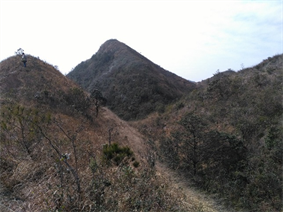Figure 1. Design cave diagram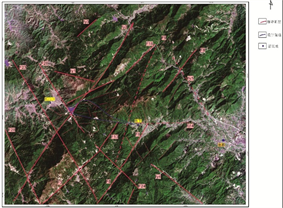Figure 2. Tectonic remote sensing interpretation

3. 现场地应力实测分析研究Table 1. CSZK14 granite section ground stress test situationTable 2. XSZK21 porphyritic section ground stress test situation

$\left(\begin{array}{c}{\sigma }_{{x}^{\prime }}\\ {\sigma }_{{y}^{\prime }}\\ {\sigma }_{{z}^{\prime }}\\ {\tau }_{{x}^{\prime }{y}^{\prime }}\\ {\tau }_{{y}^{\prime }{z}^{\prime }}\\ {\tau }_{{x}^{\prime }{z}^{\prime }}\end{array}\right)=\left[\begin{array}{cccccc}{l}_{1}^{2}& {m}_{1}^{2}& {n}_{1}^{2}& 2{l}_{1}{m}_{1}& 2{m}_{1}{n}_{1}& 2{l}_{1}{n}_{1}\\ {l}_{2}^{2}& {m}_{2}^{2}& {n}_{2}^{2}& 2{l}_{2}{m}_{2}& 2{m}_{2}{n}_{2}& 2{l}_{2}{n}_{2}\\ {l}_{3}^{2}& {m}_{3}^{2}& {n}_{3}^{2}& 2{l}_{3}{m}_{3}& 2{m}_{3}{n}_{3}& 2{l}_{3}{n}_{3}\\ {l}_{1}{l}_{2}& {m}_{1}{m}_{2}& {n}_{1}{n}_{2}& {l}_{1}{m}_{2}+{l}_{2}{m}_{1}& {m}_{1}{n}_{2}+{m}_{2}{n}_{1}& {l}_{2}{n}_{1}+{l}_{1}{n}_{2}\\ {l}_{2}{l}_{3}& {m}_{2}{m}_{3}& {n}_{2}{n}_{3}& {l}_{2}{m}_{3}+{l}_{3}{m}_{2}& {m}_{2}{n}_{3}+{m}_{3}{n}_{2}& {l}_{3}{n}_{2}+{l}_{2}{n}_{3}\\ {l}_{3}{l}_{1}& {m}_{3}{m}_{1}& {n}_{3}{n}_{1}& {l}_{3}{m}_{1}+{l}_{1}{m}_{3}& {m}_{3}{n}_{1}+{m}_{1}{n}_{3}& {l}_{3}{n}_{1}+{l}_{1}{n}_{3}\end{array}\right]\left(\begin{array}{c}{\sigma }_{x}\\ {\sigma }_{y}\\ {\sigma }_{z}\\ {\tau }_{xy}\\ {\tau }_{yz}\\ {\tau }_{xz}\end{array}\right)$ (1)Table 4. In-situ ground stress conversion value

4. 鸿图嶂隧道初始应力场回归分析

1) 依据工程勘察建立ANSYS计算模型；

2) 分别施加自重应力场及五种构造应力场边界条件，提取六次模拟计算数据，将钻孔CSZK14测点数据将其作为已知数据基于多元回归方法建立多元回归方程。将钻孔XSZK21数据代入验证多元回归效果；

3) 基于最小二乘法原理，对残差平方和进行最小化，即可获得多元回归方程中各自变量(待定因素)系数的最优解，进一步可求得鸿图嶂隧道工程区的初始地应力场 。

4.1. 数值模型建立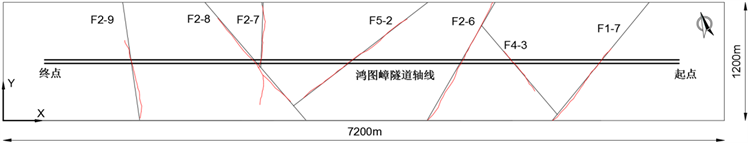Figure 3. Fault approximation processing map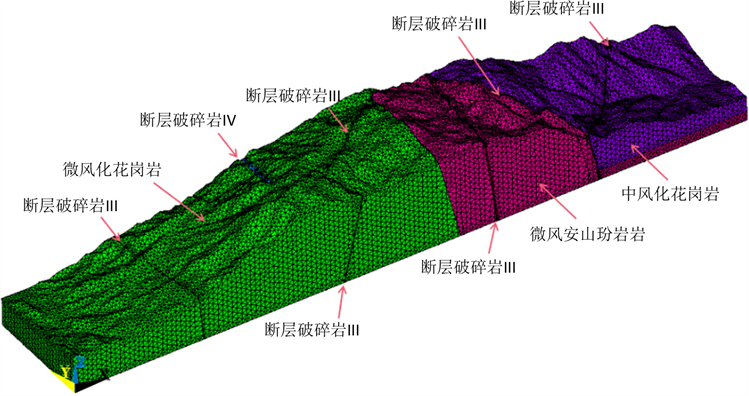Figure 4. Schematic diagram of grid division

Table5. Calculation parameters selection table

4.2. 边界条件及分析原理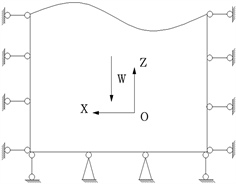(a) 自重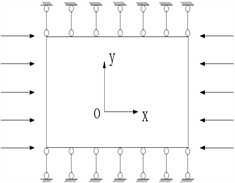(b) 东西向均匀挤压构造(c) 南北向均匀挤压构造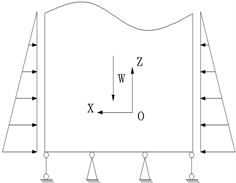(d) 东西向三角形荷载构造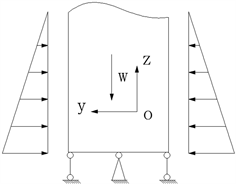(e) 南北向三角形荷载构造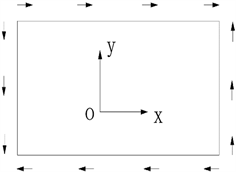(f) 水平面均匀剪切构造运动

Figure 5. Calculation of boundary conditions

1) 自重应力场：在模型的四周及底面均加上约束，将通过表5岩体参数求得的不同岩石自重应力加于模型之上。

2) 构造应力场：均匀挤压构造及三角形荷载构造通过在底部施加约束后，于两侧施加约束，另两侧施加均布(三角形)荷载实现，剪切构造运动的实现采用模拟边界位移的方式，其中于长边界(与隧道轴线方向一致的边界)上施加0.012 m位移，在短边界(垂直于轴线方向的边界)上施加0.072 m位移。

$\left({y}_{i},{x}_{i1},{x}_{i2},\cdots ,{x}_{ip}\right),\text{\hspace{0.17em}}\text{\hspace{0.17em}}i=1,2,\cdots ,N$ (2)

$Y=X\beta +\epsilon$ (3)

$R=\sqrt{\frac{{S}_{R}^{2}}{{S}_{T}^{2}}}=\sqrt{\frac{\underset{i=1}{\overset{N}{\sum }}{\left({\stackrel{^}{y}}_{i}-\stackrel{¯}{y}\right)}^{2}}{\underset{i=1}{\overset{N}{\sum }}{\left({y}_{i}-\stackrel{¯}{y}\right)}^{2}}}$ (4)

${V}_{i}=\sqrt{1-\frac{{S}_{c}}{{{S}^{\prime }}_{c}}}$ (5)

4.3. 回归结果分析

$\left[\begin{array}{cccc}\underset{k=1}{\overset{m}{\sum }}\underset{j=1}{\overset{6}{\sum }}{\left({\sigma }_{jk}^{1}\right)}^{2}& \underset{k=1}{\overset{m}{\sum }}\underset{j=1}{\overset{6}{\sum }}{\sigma }_{jk}^{1}{\sigma }_{jk}^{2}& \cdots & \underset{k=1}{\overset{m}{\sum }}\underset{j=1}{\overset{6}{\sum }}{\sigma }_{jk}^{1}{\sigma }_{jk}^{n}\\ 对& \underset{k=1}{\overset{m}{\sum }}\underset{j=1}{\overset{6}{\sum }}{\left({\sigma }_{jk}^{2}\right)}^{2}& \cdots & \underset{k=1}{\overset{m}{\sum }}\underset{j=1}{\overset{6}{\sum }}{\sigma }_{jk}^{2}{\sigma }_{jk}^{n}\\ & ⋮& ⋮& ⋮\\ & 称& & \underset{k=1}{\overset{m}{\sum }}\underset{j=1}{\overset{6}{\sum }}{\left({\sigma }_{jk}^{n}\right)}^{2}\end{array}\right]×\left[\begin{array}{c}{b}_{1}\\ {b}_{2}\\ ⋮\\ {b}_{n}\end{array}\right]=\left[\begin{array}{c}\underset{k=1}{\overset{m}{\sum }}\underset{j=1}{\overset{6}{\sum }}{\sigma }_{jk}^{1}{\sigma }_{jk}\\ \underset{k=1}{\overset{m}{\sum }}\underset{j=1}{\overset{6}{\sum }}{\sigma }_{jk}^{2}{\sigma }_{jk}\\ ⋮\\ \underset{k=1}{\overset{m}{\sum }}\underset{j=1}{\overset{6}{\sum }}{\sigma }_{jk}^{n}{\sigma }_{jk}\end{array}\right]$ (6)

$\sigma =1.2466{\sigma }_{自}+49.0772{\sigma }_{构1}+45.9447{\sigma }_{构2}-6.6716{\sigma }_{构3}-10.9647{\sigma }_{构4}+175.5855{\sigma }_{构5}$ (7)

$\sigma =1.3519{\sigma }_{自}+40.9670{\sigma }_{构1}+44.2400{\sigma }_{构2}-10.5817{\sigma }_{构3}+173.6971{\sigma }_{构5}$ (8)Table 6. Comparison table of main stress inversion of drill hole CSZK14Table 7. Validation table of main stress inversion of drill hole XSZK21

5. 鸿图嶂隧道轴线地应力分布规律研究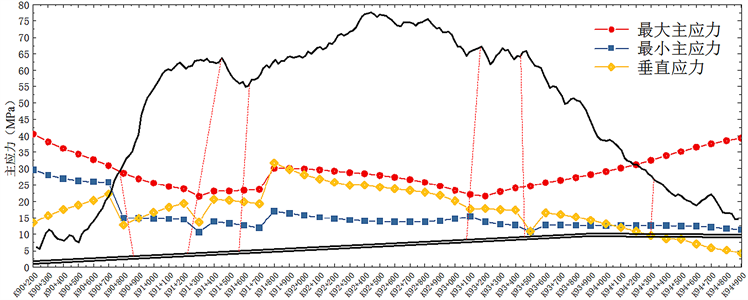Figure 6. Main stress map from K90+200 to K94+900 at the axis of Hongtuzhang Roach Tunnel

1) 鸿图嶂隧道轴线方向最大水平主应力在21 MPa以上，最大达40 MPa，根据地勘资料CSZK14钻孔岩芯饱和抗压强度90 Mpa，XSZK21钻孔岩芯饱和抗压强度80 Mpa，因此岩石强度比为2~4.29，可以判定鸿图嶂隧道为高地应力隧道。

2) 从现场实测的地应力点可以看出，鸿图嶂隧道区测点附近地应力场的分布基本以构造应力为主，在测量深度范围内三个主应力之间的大小关系依次为 ${S}_{H}>{S}_{v}>{S}_{h}$

3) 从图中隧道低埋深区域可以发现其垂直应力远低于水平主应力的范围，说明此区域影响地应力的主要因素为构造应力。

4) 从隧道中部区域的曲线走势可以发现随着隧道埋深的增大，水平主应力和垂直应力均有不同程度的增加，其中垂直应力较水平主应力增幅更大，量值上接近最大主应力，并于K91+800附近超过最大主应力，说明在中部高埋深区域，构造应力影响不强，与垂直应力在同一量值水平；

5) 隧道全线的最小水平主应力较为稳定。

6) 从图中曲线走向和断层位置对比中可以明显发现，由于断层的影响，应力场在单个断层附近出现突变，产生了一定的应力释放现象。

7) 由于部分区域断层密集存在使得该区域的最大水平主应力要明显小于周边区域。相较于独立断层处的应力突变现象也更为明显。

6. 结语

1) 通过水压致裂法原位地应力测试工作，从现场实测的地应力点可以看出，鸿图嶂隧道区测点附近地应力场的分布基本以构造应力为主，在测量深度范围内三个主应力之间的大小关系依次为 ${S}_{H}>{S}_{v}>{S}_{h}$

2) 根据已有钻孔求得的鸿图嶂隧道工程区岩体的初始应力场回归公式经验证误差较小，其结果合理可信。

3) 随着隧道埋深的增大，水平主应力和垂直应力均有不同程度的增加，其中垂直应力较水平主应力增幅更大，量值上接近最大主应力，且在断层处出现应力场突变现象。并且由于部分区域断层密集存在使得该区域的最大水平主应力要明显小于周边区域。

 代聪, 何川, 陈子全, 汪耀, 杨文波. 超大埋深特长公路隧道初始地应力场反演分析[J]. 中国公路学报, 2017, 30(10): 100-108.

 汪波, 何川, 吴德兴, 等. 苍岭特长公路隧道地应力场反演分析[J]. 岩土力学, 2012, 33(2): 629-634.

 王庆武, 巨能攀, 黄健, 杜玲丽, 张陈羊. 桑珠岭特长隧道初始地应力场反演分析[J]. 科学技术与工程, 2016, 16(25): 137-143.

 蒙伟, 何川, 张钧博, 周子寒, 汪波. 高地温高地应力下岩体初始地应力场反演分析[J]. 岩石力学与工程学报, 2020, 39(4): 749-760.

 广东省交通规划设计研究院股份有限公司. 鸿图嶂隧道设计说明[R]. 广州: 广东省交通规划设计研究院股份有限公司, 2017.

 吴俊峰, 罗永红, 周亮亮, 郭剑锋. 西南某水电站三维地应力场分布规律研究[J]. 水利水电技术, 2019, 50(7): 181-188.

 广东省交通规划设计研究院股份有限公司. 鸿图嶂隧道地应力测试[R]. 广州: 广东省交通规划设计研究院股份有限公司, 2017.

 张开俊. 初始地应力和地层参数反演分析法的研究现状与发展[J]. 水利水电技术, 1991(7): 35-39.

 田勇, 俞然刚, 张能, 张艳美. 基于ANSYS软件地应力反演的数值实验技术[J]. 实验技术与管理, 2019, 36(2): 168-170.

 杨林德. 岩土工程问题的反演理论与工程实践[M]. 北京: 科学出版社, 1996.

 王成虎, 邢博瑞, 陈永前. 长大深埋隧道工程区地应力状态预测与实例分析[J]. 岩土工程学报, 2014, 36(5): 955-960.

Top